# Subtracting Mixed NumbersExample 1: As part of his marathon training, Carlos ran three and one-fourth miles yesterday and one and three-fourths miles the day before. How much farther did Carlos run yesterday than the day before?

Analysis: This problem is asking us to subtract mixed numbers with like denominators.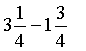In the last lesson, we learned that to add mixed numbers, add the whole numbers and add the fractions separately: (whole + whole) + (fraction + fraction). A similar procedure applies to subtracting mixed numbers. However, how do we subtract three-fourths from one-fourth? In order to subtract a larger unit from a smaller, we will need to borrow. For example, if you were subtracting 31-19, you would borrow one ten and then regroup it as 10 ones in order to subtract. Recall that a mixed number consists of a whole-number part and a fractional part. Let's use this fact and fraction circles to help us convert one whole into 4 fourths so we can regroup and borrow.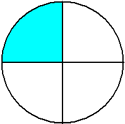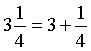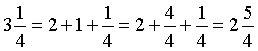Now that we have rewritten three and one-fourth as two and five-fourths, we can subtract these mixed numbers.Example 1: As part of his marathon training, Carlos ran three and one-fourth miles yesterday and one and three-fourths miles the day before. How much farther did Carlos run yesterday than the day before?

Analysis: This problem is asking us to subtract mixed numbers with like denominators. We need to regroup and borrow.Let's look at some more examples.

Example 2: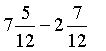Analysis: These mixed numbers have like denominators. In order to subtract a larger unit from a smaller one, we will need to borrow.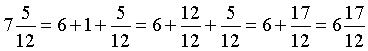Solution: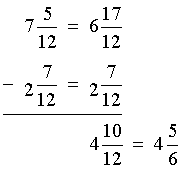Example 3:Analysis: We are subtracting a whole number from a mixed number.

Solution: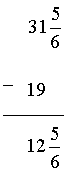Example 4: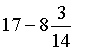Analysis: We are subtracting a mixed number from a whole number. We will need to borrow.Solution:Example 5: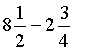Analysis: The fractional parts have unlike denominators.

Step 1: We will write equivalent fractions using the LCD, 4.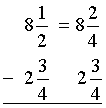Step 2: We will need to borrow.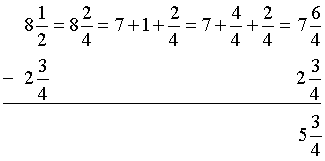Example 6: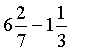Analysis: The fractional parts have unlike denominators.

Step 1: We will write equivalent fractions using the LCD, 21.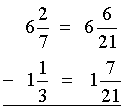Step 2: We will need to borrow.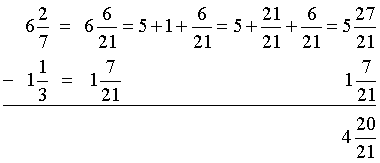Example 7:Analysis: The fractional parts have unlike denominators.

Step 1: We will write equivalent fractions using the LCD, 12.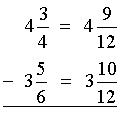Step 2: We will need to borrow.It is important to note that another way to subtract mixed numbers is to convert each mixed number into an improper fraction. For example 7, this would be done as follows: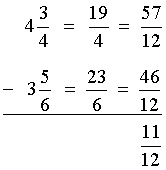This method is mathematically sound. However, it can lead to careless arithmetic errors, so we do not recommend it.Example 8: At the end of a football game, the head coach noticed that the jug of water, which had initially contained nine and three-eighths liters, was down to three and nine-sixteenths liters. How many liters of water was consumed?

Analysis: This problem is asking us to subtract the following mixed numbers: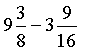Solution: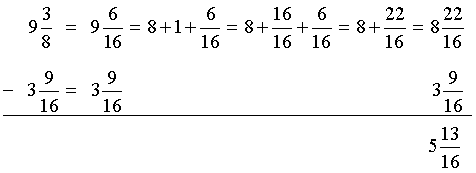Five and thirteen-sixteenths liters of water was consumed.

Summary: To subtract mixed numbers:

1. If the denominators are unlike, use the LCD to rewrite them as equivalent fractions.
2. If the second fraction is larger than the first, borrow a whole number and convert it to an equivalent fraction using the LCD.
3. Subtract the whole numbers and subtract the fractions separately: (whole - whole) + (fraction - fraction)
4. Simplify the result, if necessary.

### Exercises

Directions: Subtract the mixed numbers in each exercise below. Be sure to simplify your result, if necessary. Click once in an ANSWER BOX and type in your answer; then click ENTER. After you click ENTER, a message will appear in the RESULTS BOX to indicate whether your answer is correct or incorrect. To start over, click CLEAR.

Note: To write the mixed number four and two-thirds, enter 4, a space, and then 2/3 into the form.

 1.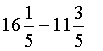ANSWER BOX:   RESULTS BOX:
 2.ANSWER BOX:   RESULTS BOX:
 3.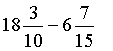ANSWER BOX:   RESULTS BOX:
 4. A painter had twenty and one-fourth gallons of paint in her bucket at the beginning of the day, and only nine and three-eighths gallons at the end of the day. How many gallons of paint did she use? ANSWER BOX:   gallons   RESULTS BOX:
 5. A technology stock opened at thirty-one and three-eighths and closed at twenty-seven and nine-sixteenths. What was the net loss for this stock? ANSWER BOX:     RESULTS BOX:
 Lessons on Adding and Subtracting Fractions and Mixed Numbers 1. Adding Fractions with Like Denominators 2. Subtracting Fractions with Like Denominators 3. Adding and Subtracting Fractions with Unlike Denominators 4. Adding Mixed Numbers 5. Subtracting Mixed Numbers 6. Solving Word Problems 7. Practice Exercises 8. Challenge Exercises 9. Solutions